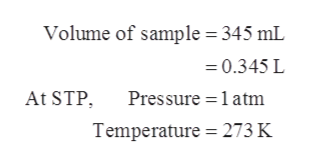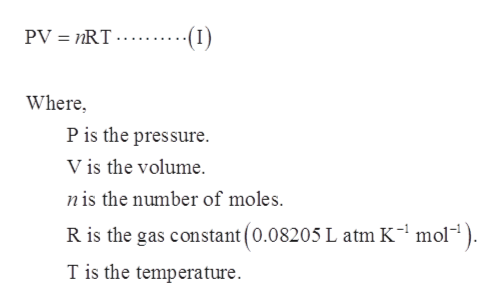# A sample of Argon gas is allowed to adjust to STP. If the sample has a volume of 345 mL, what is the mass of the sample?

Question
32 views

A sample of Argon gas is allowed to adjust to STP. If the sample has a volume of 345 mL, what is the mass of the sample?

check_circle

Step 1

Ideal gas equation is also known as gas density equation. The ideal gas equation is shown below.

Step 2

Given data:help_outlineImage TranscriptioncloseVolume of sample 345 mL 0.345 L At STP Pressure 1atm Temperature 273 K fullscreen
Step 3

The number of moles of argon is calculated by u...help_outlineImage TranscriptionclosePV nRT (I) Where P is the pressure. Vis the volume. nis the number of moles. R is the gas constant (0.08205 L atm K1 mol1). T is the temperature fullscreen

### Want to see the full answer?

See Solution

#### Want to see this answer and more?

Solutions are written by subject experts who are available 24/7. Questions are typically answered within 1 hour.*

See Solution
*Response times may vary by subject and question.
Tagged in

### Chemistry